Courses

# Narrowband Noise Representation Electronics and Communication Engineering (ECE) Notes | EduRev

## Electronics and Communication Engineering (ECE) : Narrowband Noise Representation Electronics and Communication Engineering (ECE) Notes | EduRev

The document Narrowband Noise Representation Electronics and Communication Engineering (ECE) Notes | EduRev is a part of Electronics and Communication Engineering (ECE) category.
All you need of Electronics and Communication Engineering (ECE) at this link: Electronics and Communication Engineering (ECE)

Narrowband Noise Representation

In most communication systems, we are often dealing with band-pass filtering of signals. Wideband noise will be shaped into band limited noise. If the bandwidth of the band limited noise is relatively small compared to the carrier frequency, we refer to this as narrowband noise. We can derive the power spectral density G n(f) and the auto-correlation function Rnn(τ) of the narrowband noise and use them to analyze the performance of linear systems. In practice, we often deal with mixing (multiplication), which is a non-linear operation, and the system analysis becomes difficult. In such a case, it is useful to express the narrowband noise as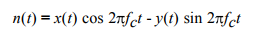(1)

where fc is the carrier frequency within the band occupied by the noise. x(t) and y(t) are known as the quadrature components of the noise n(t). The Hibert transform of n(t) is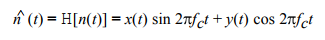Proof:

The Fourier transform of n (t) is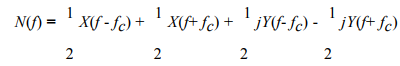Let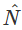(f) be the Fourier transform of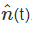. In the frequency domain,(f) = N(f)[-j sgn(f)]. We simply multiply all positive frequency components of N(f) by -j and all negative frequency components of N(f) by j. Thus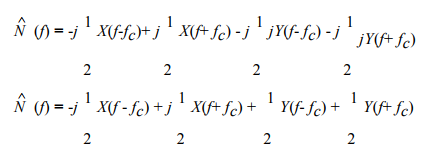and the inverse Fourier transform of(f) is= x(t) sin2πfc t + y(t) cos2πfct

The quadrature components x(t) and y(t) can now be derived from equations (1) and (2).

x(t) = n(t)cos 2πfct +sin 2πfct                (3)

and

y(t) = n(t)cos 2πfct-sin 2πfct                   (4)

Given n(t), the quadrature components x(t) and y(t) can be obtained by using the arrangement.

x(t) and y(t) have the following properties:

1. E[x(t) y(t)] = 0. x(t) and y(t) are uncorrelated with each other.

2. x(t) and y(t) have the same means and variances as n(t).

3. If n(t) is Gaussian, then x(t) and y(t) are also Gaussian.

4. x(t) and y(t) have identical power spectral densities, related to the power spectral density of n(t) by

Gx(f) = Gy(f) = Gn(f- fc) + Gn(f+ fc )                (5)

for fc - 0.5B < | f | < fc + 0.5B and B is the bandwidth of n(t).

Proof:

Equation (5) is the key that will enable us to calculate the effect of noise on AM and FM systems. It implies that the power spectral density of x(t) and y(t) can be found by shifting the positive portion and negative portion of Gn(f) to zero frequency and adding to give Gx (f) and Gy (f).

In the special case where Gn(f) is symmetrical about the carrier frequency fc , the positive- and negative-frequency contributions are shifted to zero frequency and added to give

G(f) = Gy(f) = 2Gn(f- fc ) = 2Gn(f+ fc )

Performance of Binary FSK:

Consider the synchronous detector of binary FSK signals. In the presence of additive white Gaussian noise (AWGN), w(t), the received signal is

r(t) = Acos 2 fc1 t + w(t)

where A is a constant and fc1 is the carrier frequency employed if a 1 has been sent. The signals at the output of the band-pass filters of centre frequencies fc1 and fc2 are

r1 (t) = Acos 2 fc1 t + n1 (t)

and

r(t) = n2 (t)

where n1 (t) = x1 (t) cos 2 fc1 t - y1 (t) sin 2 fc1 t

and n2 (t) = x2 (t) cos 2 fc2 t - y2 (t) sin 2 fc2t

are the narrowband noise.

With appropriate design of low-pass filter and sampling period, the sampled output signals are

vo1 = A + x

vo2 = x2

and

v = A + [x1 - x2 ].

x1 and x2 are statistically independent Gaussian random variables with zero mean and fixed variance σ= N, where N is the power of the random variable. It can be seen that one of the detectors has signal plus noise, the other detector has noise only.

When fc2 is the carrier frequency employed for sending a 0, the received signal is r(t) = Acos 2 fc2 t + w(t). It can be

shown that

v = -A + [x1 - x2 ]

Since E [x1 - x2 ]2 = E [x 1 ]2 - 2E [x 1 x2 ]2 + E [x]2 = E [x1 ]2 + E [x2]2 = 2 + 2, the total

variance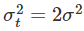Offer running on EduRev: Apply code STAYHOME200 to get INR 200 off on our premium plan EduRev Infinity!

,

,

,

,

,

,

,

,

,

,

,

,

,

,

,

,

,

,

,

,

,

;